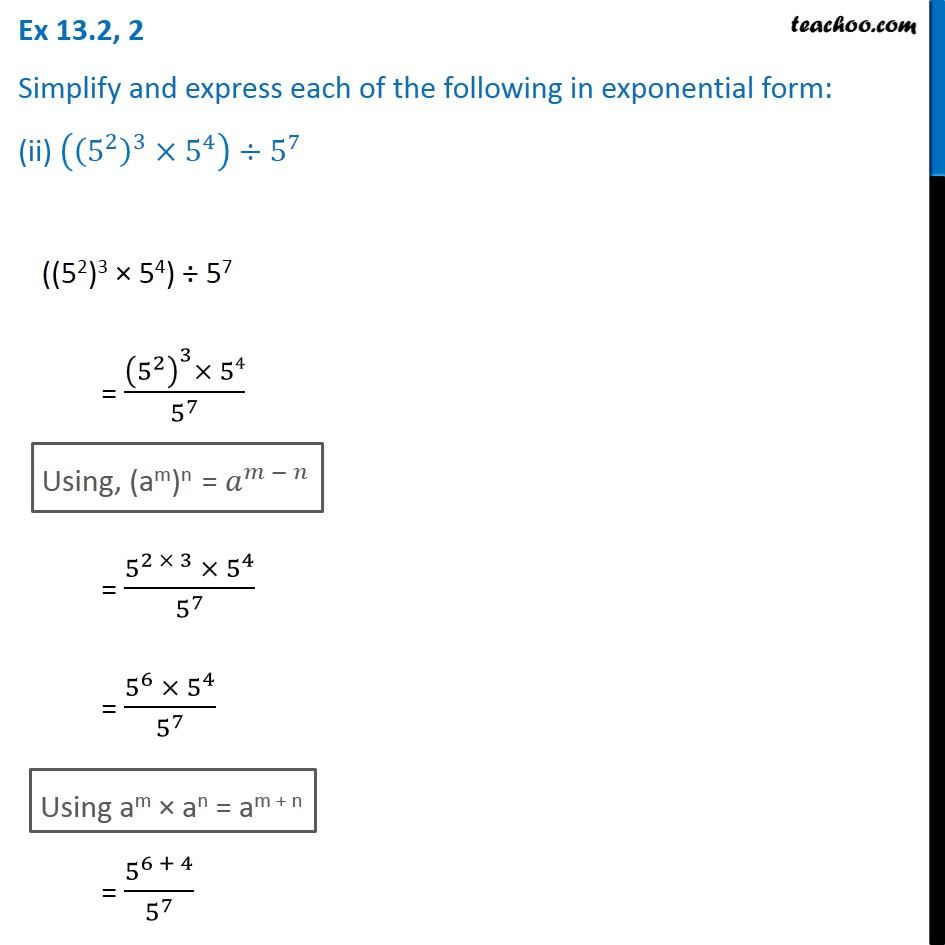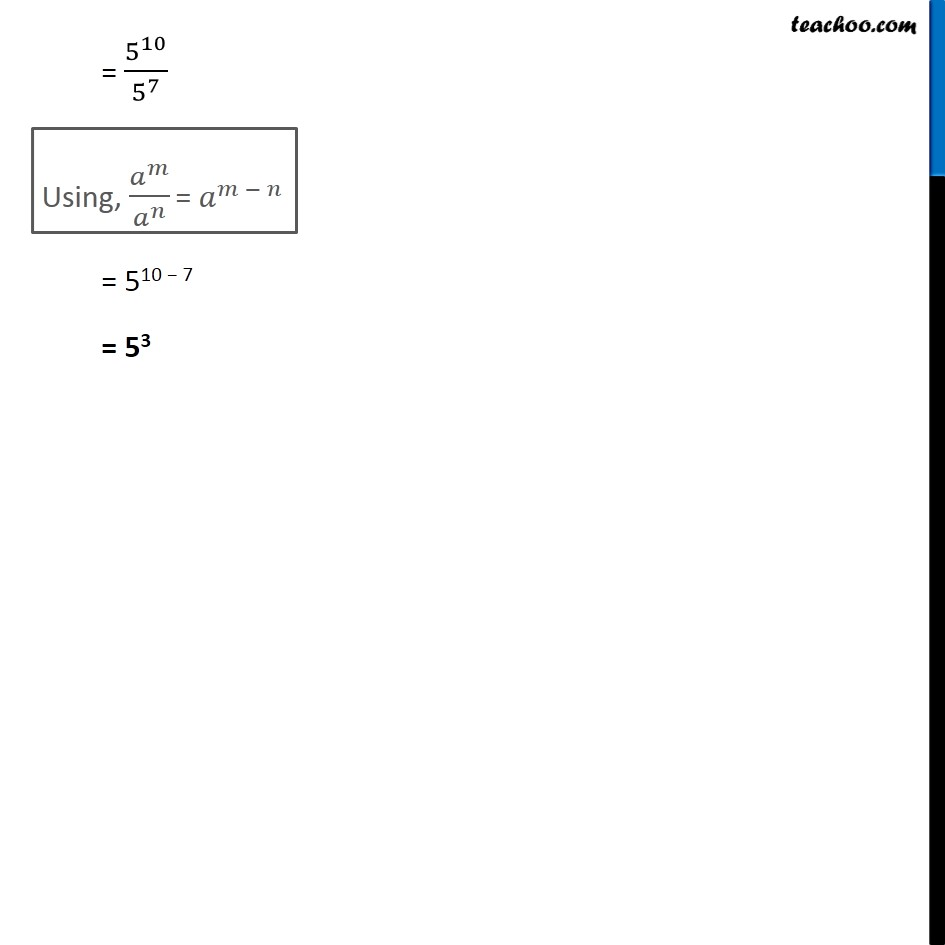1. Chapter 13 Class 7 Exponents and Powers
2. Serial order wise
3. Ex 13.2

Transcript

Ex 13.2, 2 Simplify and express each of the following in exponential form: (ii) ((5^2 )^3×5^4 )÷5^7 ((52)3 × 54) ÷ 57 = ((5^2 )^3× 54)/5^7 Using, (am)n = 𝑎^(𝑚 − 𝑛) = (5^(2 × 3) × 5^4)/5^7 = (5^6 × 5^4)/5^7 Using am × an = am + n = 5^(6 + 4)/5^7 = 5^10/5^7 Using, 𝑎^𝑚/𝑎^𝑛 = 𝑎^(𝑚 − 𝑛) = 510 − 7 = 53